# Texas Go Math Grade 5 Lesson 17.4 Answer Key Paying Bills

Refer to our Texas Go Math Grade 5 Answer Key Pdf to score good marks in the exams. Test yourself by practicing the problems from Texas Go Math Grade 5 Lesson 17.4 Answer Key Paying Bills.

## Texas Go Math Grade 5 Lesson 17.4 Answer Key Paying Bills

Unlock the Problem.

Mrs. Pastella wants to buy an easel for $100 at a store. She has no cash with her. Complete the table below to help her determine the method of payment she should use. Write the letter of the statements to show the advantages and disadvantages of each method. A statement can go in more than one place.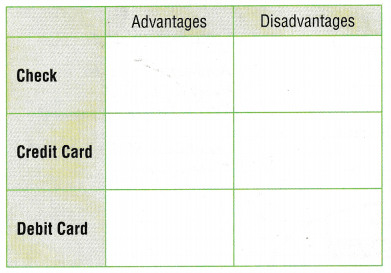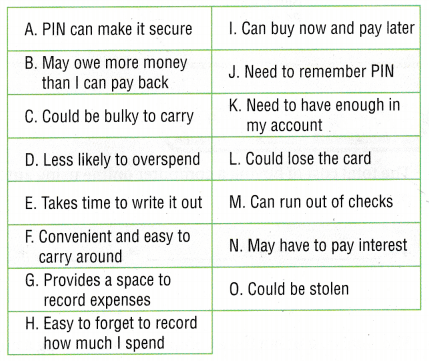Answer: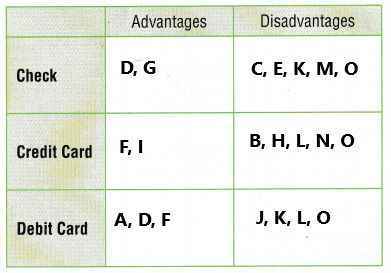Example 1 Sometimes it is not possible to use cash or a check. In such cases, you can use a credit card or one of several methods of electronic payment. These methods are especially useful when you shop online. Some companies offer money transfer services to send money immediately from your account to the seller. There is usually a fee for the service. Some sellers let you pay the cost in several parts. You set up automatic payments that transfer a set amount of money from your account at specific times. You usually pay a fee for spreading out the payments. Automatic payments are also useful for bills that occur regularly. Write the letter of the statements to show the advantages and disadvantages of each method of payment. A statement can go in more than one place.Answer:Question 1. The total cost of buying a computer online using automatic payments: 4 × ___ + 4 × ___ = ____ + ____ = _____ Answer: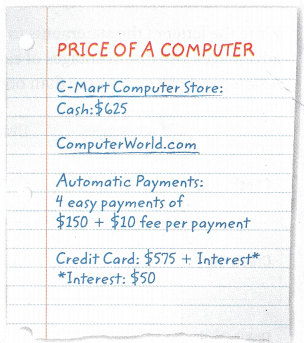The total cost of buying a computer online using automatic payments: 4 x$150 + 4 x $10 =$600 + $40 =$640
Explanation:
In the above image we can observe the price of a computer. We can buy a computer with different payments like cash, automatic payments, credit card. Here we buying a computer online using automatic payments. The four easy payments of $150 plus$10 fee per payment. Multiply 4 with $150 then the product is$600. Multiply 4 with $10 the product is$40. Add $600 with$40 then the sum is $640. The total cost of buying a computer online using automatic payments is$640.

Texas Go Math Grade 5 Answer Key Pdf Lesson 17.4 Question 2.
The total cost of buying a computer online using a credit card:
___ + ___ = ____
The total cost of buying a computer online using a credit card:
$575 +$50 = $625 Explanation: In the above image, we can observe the price of a computer. We can buy a computer with different payments like cash, automatic payments, credit card. Here we buying a computer online using a credit card. The payment cost is$575 with interest. Here interest is $50. Add$625 with $50 the sum is$625. The total cost of buying a computer online using a credit card is $625. Problem Solving Question 3. Apply Name one method of payment that allows you to use money in your account and one method that allows you to pay the amount at a later time. Answer: Debit card is the one method of payment that allows us to use money in our account. Credit card is the one method that allows us to pay the amount at a later time. Question 4. H.O.T. Analyze What are the advantages and disadvantages of the methods you chose in Exercise 3? Answer: Advantages of debit card: 1. we don’t have to carry cash with us. Cash isn’t easy to track. 2. Debit cards are widely accepted around the world. 3. Multiple debit cards can be associated with one account. 4. A debit card is issued by a bank that allows a user to immediately use money from an account. Disadvantages of debit card: 1. No credit is allowed A debit card is linked to our bank account. There is no possibility of making any transaction on credit. 2. There can be several fees associated with your debit card activities. 3. If we forget our PIN, then we might not have the option to complete a transaction. 4. Debit card rewards are often limited in size and availability. Advantages of credit card: 1. The biggest advantage of a credit card is its easy access to credit. 2. EMI facility If we plan on making a large purchase and don’t want to sink our savings into it, we can choose to put it on our credit card. Disadvantages of credit card: 1. The biggest con of a credit card is the minimum due amount that is displayed at the top of a bill statement. 2. High interest rate. If we do not clear our dues by our billing due date, the amount is carried forward and interest is charged on it. Problem Solving Question 5. Multi-Step Ty researches how much he would pay if he used various methods of payment to buy a chemistry set. If he pays$0.10 in interest for every dollar on his credit card, which method should he use to buy the chemistry set? Explain.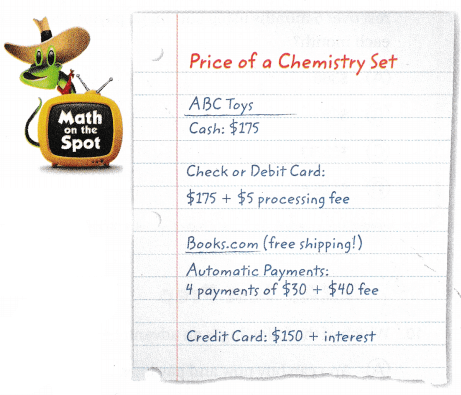Cash is $175. Check or debit card is$175 + $5 processing fee.$175 + $5 =$ 180
Check or debit card payment method is $180. Automatic payments is 4 payments of$30 + $40 fee. 4($30 + $40 ) = 4($70) = $280 Automatic payment method is$280.
He pays $0.10 in interest for every dollar on his credit card. Credit card is$150 + interest
Interest is $150 x$0.01 = $15$150 +$15 =$165
Credit payment method is $165. Credit card payment method is used to buy the chemistry set. Explanation: In the above image we can observe a price of chemistry set. In that image different types of payment methods like cash, Check or debit card, Automatic payments, credit card. Cash is$175. Check or debit card is $175 +$5 processing fee. Add $175 with$5 the sum is $180. Check or debit card payment method is$180.
Automatic payments is 4 payments of $30 +$40 fee. Add $30 with$40 the sum is $70. Multiply$70 with 4 the product is $280. Automatic payment method is$280. He pays $0.10 in interest for every dollar on his credit card. Credit card is$150 + interest. Interest is calculated by multiplying $150 with$0.01 the product is $15. Add$150 with $15 the sum is$165. Credit payment method is $165. Credit card payment method is used to buy the chemistry set. Question 6. Liam’s bank pays the amount on a check five days after it is written. If Liam wants to make a payment immediately, which method of payment could he use? Explain. Answer: Daily Assessment Task Fill in the bubble completely to show your answer. Question 7. Jen wants to buy a camera. Which method will let her buy now and pay later? (A) check (B) cash (C) credit card (D) money transfer Answer: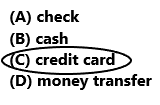option C is correct. Explanation: Jen wants to buy a camera. If he buys a camera with a credit card he can buy the camera now and pay later. Lesson 17.4 5th Grade Go Math Answer Key Question 8. Antoine owes S684. He can make six monthly automatic payments with a$2.50 fee for each payment, or he can pay using a credit card. He will pay $30 in interest if he uses his credit card. How much money does he save by using automatic payments? (A)$15
(B) $114 (C)$116.50
(D) $16 Answer:Antoine owes$684. He can make six monthly automatic payments with a $2.50 fee for each payment.$684 + $2.50 x 6$684 + $15$699
The automatic payment is $699. If he uses his credit card, he will pay$30 in interest.
$684 +$30 = $714 The credit card payment is$714.
$714 –$699 = $15 By using automatic payments he saved$15.
So, option A is correct.
Explanation:
Antoine owes $684. He can make six monthly automatic payments with a$2.50 fee for each payment. Multiply $2.50 with 6 months the product is$15. Add $15 with$684 the sum is $699. The automatic payment is$699. He will pay $30 in interest if he uses his credit card. Add$684 with $30 the sum is$714. The credit card payment is $714. Subtract$699 from $714 the difference is$15. By using automatic payments he saved $15. So, option A is correct. Question 9. Multi-Step Lester buys a sofa for$560. He pays $200 first and pays the rest over 6 months using automatic payments. How much will he pay each month? (A)$360
(B) $60 (C)$93.33
(D) $126.67 Answer:Lester buys a sofa for$560.
He pays $200 first.$560 – $200 =$360
He pays the rest over 6 months using automatic payments.
$360/6 =$60
He pay $60 for each month. So, Option B is correct. Explanation: Lester buys a sofa for$560. He pays $200 first and pays the rest over 6 months using automatic payments. Subtract$200 from $560 the difference is$360. Next divide $360 with 6 months the quotient is$60. He pay $60 for each month. So, Option B is correct. Texas Test Prep Question 10. Which of the following is an advantage of using a check to pay bills? (A) You can buy now and pay later. (B) The money is transferred immediately. (C) You can pay using money in your account. (D) You may have to pay interest on the amount. Answer: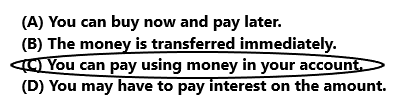Option C is correct. Explanation: The advantage of using a check to pay bills is we can pay using money in our account. So option C is correct. ### Texas Go Math Grade 5 Lesson 17.4 Homework and Practice Answer Key Angelina made a chart to compare the cost of buying a game console using different payment methods. Use the chart for 1-4. Question 1. The total cost of buying a game console at Ed’s Discount Store is ____. Answer: The total cost of buying a game console at Ed’s Discount Store is$229.
Explanation:
In the below image we can observe a price of a game console data. In that data we can observe Ed’s Discount store is $229. The total cost of buying a game console at Ed’s Discount Store is$229.

Question 2.
The total cost of buying a game console online using automatic payments: ___ × (___ + ___) = ___.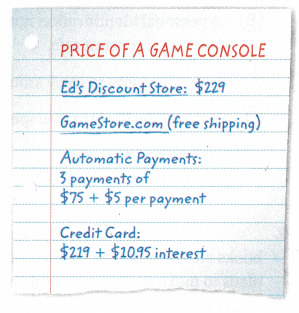Buying a game console online using automatic payments.
3 x ($75 +$5)
3 x $80$240
The total cost of buying a game console online using automatic payments is $240. Explanation: In the above image we can observe a price of a game console data. In that image we can observe automatic payments. Buying a game console online using automatic payments is 3 payments of$75 plus 45 per payment. First add $75 with$5 the sum is $80. Multiply 3 with$80 then the product is $240. The total cost of buying a game console online using automatic payments is$240.

The total cost of buying a game console using a credit card: __ + ___ = ___
$219 +$10.95 = $229.95 The total cost of buying a game console using a credit card is$229.95.
Explanation:
In the above image we can observe a price of a game console data. In that image we can observe automatic payments. Buying a game console using credit card is $219 plus$10.95 interest. Add $219 with$10.95 then the sum is $229.95. The total cost of buying a game console using a credit card is$229.95.

Question 4.
Why might Angelina choose to purchase the game console using a more expensive payment method?
Angelina may not have the cash, She may not have the enough available credit on her or need to save it for something. For this reason Angelina choose to purchase the game console using a more expensive payment method.

Problem Solving

Question 5.
Jada researches various methods of pa3ment to buy a video game. If Jada pays $0.03 in interest for every dollar on her credit card, which method will be the least expensive? Which method is the most expensive? Answer:Cash payment is$49.
Check or Debit card is $49 +$5 processing fee.
$49 +$5 = $54 Automatic payments is 2 payments of$26 + $2 per payment. 2 x ($26 + $2) 2 x ($28)
$56 Automatic payments is$56.
Credit card is $48. Jada pays$0.03 in interest for every dollar on her credit card.
$1 =$0.03
$48 = ?$
$48 x$0.03 = $1.44 Credit card is$48.
$48 +$1.44 = $49.44 Credit card payment is$49.44.
Cash is least expensive $49.00. Automatic payments is more expensive$56.00.
Explanation:
In the above image we can observe a price of a video game data. In that image we can observe different payment methods are cash payment, Check or Debit card payment, automatic payments, and credit card payment. We know that Cash payment is $49. Check or Debit card payment is$49 + $5 processing fee. Add$49 with $5 the sum is$54.  Automatic payments is 2 payments of $26 +$2 per payment. Add $26 with$2 the sum is $28. Multiply$28 with 2 the product is $56. Automatic payments is$56. Credit card is $48. Jada pays$0.03 in interest for every dollar on her credit card. Multiply $48 with$0.03 the product is $1.44. Add$48 with $1.44 interest the sum is$49.44. Credit card payment is $49.44. So, Cash method is least expensive$49.00 and Automatic payments method is more expensive $56.00. Question 6. Oliver pays$0.05 interest for every $1 he spends using his credit card. Explain how he can use mental math to find the total cost of a$300 tablet computer.
Oliver pays $0.05 interest for every$1 he spends using his credit card.
$1 =$0.05 interest
The total cost of a $300 tablet computer.$300 x $0.05 =$15.00
$300 +$15.00 = $315.00 The total cost of a tablet computer is$315.00.
Explanation:
Oliver pays $0.05 interest for every$1 he spends using his credit card. The total cost of a $300 tablet computer is calculated by first multiplying$300 with $0.05 the product is$15.00. Next add $300 with$15.00 then the sum is $315.00. Lesson Check Fill in the bubble completely to show your answer. Question 7. Which of the following is NOT an advantage of using a debit card as your payment method? (A) You can buy now and pay later. (B) A personal identification number helps keep your account safe. (C) It is convenient to carry around. (D) You are less likely to overspend. Answer: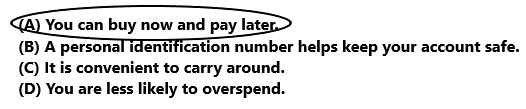Option A is correct. Explanation: Option A is correct because it is not an advantage of using a debit card as our payment method. We can buy now and pay later is used in credit card payment method. Question 8. Harrison pays$0.02 in interest for every $1 that he spends on his credit card. How much interest will Harrison pay on the purchase of a$78 camera bag?
(A) $1.40 (B)$1.56
(C) $15.60 (D)$79.56$78 x$0.02 = $1.56 Harrison has to pay the interest$1.56 on the purchase of a $78 camera bag. So, Option B is correct. Explanation: Harrison pays$0.02 in interest for every $1 that he spends on his credit card. We have to calculate the interest on the purchase of a$78 camera bag. Multiply $78 with$0.02 the product is $1.56. Harrison has to pay the interest$1.56 on the purchase of a $78 camera bag. So, Option B is correct. Go Math Answer Key 5th Grade Lesson 17.4 Question 9. Multi-Step Charlotte wants to buy some books online that have a total cost of$162. She plans to make six equal monthly payments. Each month, she will be charged a $1.75 processing fee. How much will Charlotte pay each month? (A)$27.00
(B) $25.25 (C)$28.75
(D) $27.75 Answer: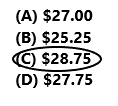$162/6 = $27$27 + $1.75 =$28.75
Charlotte pay $28.75 each month. So, option C is correct. Explanation: Charlotte wants to buy some books online that have a total cost of$162. She plans to make six equal monthly payments. Each month, she will be charged a $1.75 processing fee. First divide$162 by 6 months the quotient is $27. Add$27 with $1.75 the sum is$28.75. Charlotte pay $28.75 each month. So, option C is correct. Question 10. Multi-Step Ethan wants to buy a new watch that costs$112. He pays $0.04 in interest for every$1 that he spends on his credit card. How much will Ethan save by paying with cash?
(A) $2.80 (B)$1.04
(C) $0.45 (D)$4.48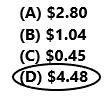$112 x$0.04 = $4.48 He has to pay interest$4.48 to buy a new watch on his credit card.
Ethan save $4.48 by paying with cash. So, option D is correct. Explanation: Ethan wants to buy a new watch that costs$112. He pays $0.04 in interest for every$1 that he spends on his credit card. Multiply $112 with$0.04 the product is $4.48. He has to pay interest$4.48 to buy a new watch on his credit card. Ethan save $4.48 by paying with cash. So, option D is correct. Question 11. Multi-Step Enrique buys a jacket for$35 and pants for $29. Sales tax is$0.07 for every dollar of the purchase price. He pays a $2.75 processing fee for paying with a check. How much will Enrique pay for his purchases? (A)$71.23
(B) $68.48 (C)$71.42
(D) $66.75 Answer:Enrique buys a jacket for$35 and pants for $29.$35 + $29 =$64
Enrique buys both jacket and pants for $64. Sales tax is$0.07 for every dollar of the purchase price.
$0.07 x$64 = $4.48 Sales tax is$4.48 on Enrique purchases.
$64 +$4.48 = $68.48 He pays a$2.75 processing fee for paying with a check.
$68.48 +$2.75 = $71.23 Enrique pay$71.23 for his purchases.
So option A is correct.
Explanation:
Enrique buys a jacket for $35 and pants for$29. Add $35 with$29 the sum is $64. Enrique buys both jacket and pants for$64. Sales tax is $0.07 for every dollar of the purchase price. Multiply$0.07 with $64 the product is$4.48. Sales tax on Enrique purchases is $4.48. Add$64 with $4.48 the sum is$68.48. He pays a $2.75 processing fee for paying with a check. Add$68.48 with $2.75 the sum is$71.23. Enrique pay $71.23 for his purchases. So option A is correct. Question 12. Multi-Step Paige finds a printer for$148 at an online store. The cost of shipping the printer Is $20. If Paige plans to make four equal monthly payments for the total cost, how much will she pay each month? (A)$37
(B) $42 (C)$32
(D) $40 Answer: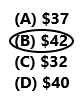$148 + $20=$168
The total cost of the printer is $168. Paige plans to make four equal monthly payments for the total cost.$168/4 = $42 Each month she pay$42.
So option B is correct.
Explanation:
Paige finds a printer for $148 at an online store. The cost of shipping the printer Is$20. Add $148 with$20 the sum is $168. The total cost of the printer is$168. Paige plans to make four equal monthly payments for the total cost. Divide $168 by 4 the quotient is$42. Each month she pay \$42. So option B is correct.

Scroll to Top